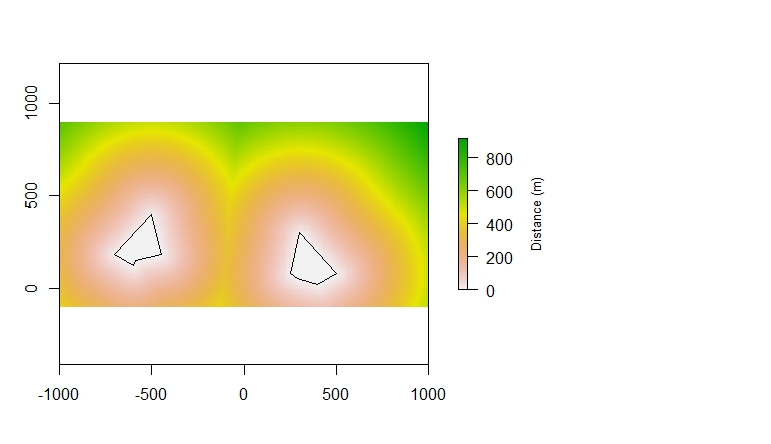There are many cases when I have needed to calculate a distance from a point or many points to a feature in GIS. Until recently I have always used the near tool. This works really well for small datasets but can take forever with larger datasets. The near calculation also only works with points (I'm sure that there is a raster equivalent I just haven't done the research on it). I also really like to document where I got my data. You can do that in ArcGIS but it is an added step to do it.

So, I'm back to R. Near calculations, or `distance()` calculations in r, are also slow, but because it is easy to loop through datasets to perform the same calculation many times, I don't mind running a program all night to calculate the distance to many objects.

There are two types of calculations that I need to perform for the analysis I'm doing. The first is a distance to polygon layers. The second is distance to line features. It appears that you perform these calculation is very different ways. We'll start with the distance to polygons because that one is a bit more strait forward although still bit confusing.

Source: I used this Stack Exchange thread for this example.

## Distance to polygon

``library(rgeos)``

Make A raster

``r<- raster(ncol=200, nrow=150, xmn=-1000, xmx=1000, ymn=-100, ymx=900)rr<-setValues(r, 0)``

Create a two polygons and merge (bind) them together.

``##Make polygon 1x<- c(300, 500, 400, 289, 250)y<- c(300, 80, 20, 50, 80)poly1<-SpatialPolygons(list(Polygons(list(Polygon(data.frame(x,y))), ID=1)))##Make polygon2x<- c(-500, -700, -600, -589, -450)y<- c(400, 180, 120, 150, 180)poly2<-SpatialPolygons(list(Polygons(list(Polygon(data.frame(x,y))), ID=1)))##Merge and Plot the polygonspoly<-bind (poly1, poly2)plot(poly)``

Mask raster by the geometry of the polygon. You need to do this because the distance calculation works by calculating the distance of each cell to the nearest NA valued cell. That is why when we made the raster we set the values of the whole raster to 0 instead of leaving them as NA `setValues(r, 0)`.

``rrr<-mask(rr, poly)``

Run the distance calculation and then plot.

``rD<- distance(rrr)plot(rD,     legend.args=list(text='Distance (m)', side=4, line=3, cex=0.8))plot(poly, add=T)``

The finished product should look something like this:## Distance to Line

I utalized this Stack Exchange Post

``library(rgeos)library(raster)``

Make the same raster that we used for the last example:

``## Make the same raster as abover<- raster(ncol=200, nrow=150, xmn=-1000, xmx=1000, ymn=-100, ymx=900)r<-setValues(r, 0)``

Create two lines together each with their own ID.

``##Make two lineslines<-SpatialLines(list(  Lines(list(Line(cbind(c(300, 500, 400, 289, 250), c(300, 80, 20, 50, 80)))), ID="a"),  Lines(list(Line(cbind(c(-500, -700, -600, -589, -450), c(400, 180, 120, 150, 180)))), ID="b")))plot(lines)``

Use gDistance from the lines to raster converted to points (this could take a long time) and use the ID to differentiate between the lines. Then add the distances from the points to the raster.

``##Calculate Distance from Raster to linesdistance<-gDistance(lines, as(r, "SpatialPoints"), byid=TRUE)r[]<-apply(distance,1, min)``

Then plot to see the result

``plot(r,     legend.args=list(text='Distance (m)', side=4, line=3, cex=0.8))plot(lines, add=T)``